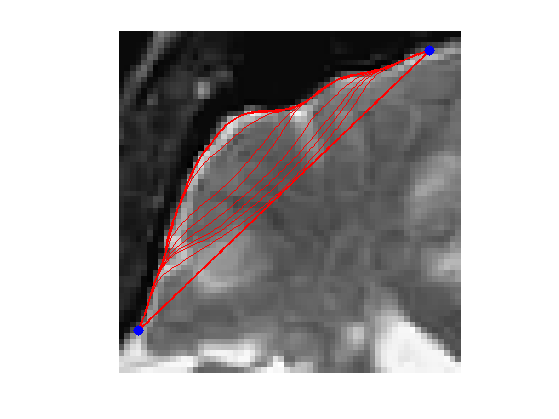$\newcommand{\NN}{\mathbb{N}} \newcommand{\CC}{\mathbb{C}} \newcommand{\GG}{\mathbb{G}} \newcommand{\LL}{\mathbb{L}} \newcommand{\PP}{\mathbb{P}} \newcommand{\QQ}{\mathbb{Q}} \newcommand{\RR}{\mathbb{R}} \newcommand{\VV}{\mathbb{V}} \newcommand{\ZZ}{\mathbb{Z}} \newcommand{\FF}{\mathbb{F}} \newcommand{\KK}{\mathbb{K}} \newcommand{\UU}{\mathbb{U}} \newcommand{\EE}{\mathbb{E}} \newcommand{\Aa}{\mathcal{A}} \newcommand{\Bb}{\mathcal{B}} \newcommand{\Cc}{\mathcal{C}} \newcommand{\Dd}{\mathcal{D}} \newcommand{\Ee}{\mathcal{E}} \newcommand{\Ff}{\mathcal{F}} \newcommand{\Gg}{\mathcal{G}} \newcommand{\Hh}{\mathcal{H}} \newcommand{\Ii}{\mathcal{I}} \newcommand{\Jj}{\mathcal{J}} \newcommand{\Kk}{\mathcal{K}} \newcommand{\Ll}{\mathcal{L}} \newcommand{\Mm}{\mathcal{M}} \newcommand{\Nn}{\mathcal{N}} \newcommand{\Oo}{\mathcal{O}} \newcommand{\Pp}{\mathcal{P}} \newcommand{\Qq}{\mathcal{Q}} \newcommand{\Rr}{\mathcal{R}} \newcommand{\Ss}{\mathcal{S}} \newcommand{\Tt}{\mathcal{T}} \newcommand{\Uu}{\mathcal{U}} \newcommand{\Vv}{\mathcal{V}} \newcommand{\Ww}{\mathcal{W}} \newcommand{\Xx}{\mathcal{X}} \newcommand{\Yy}{\mathcal{Y}} \newcommand{\Zz}{\mathcal{Z}} \newcommand{\al}{\alpha} \newcommand{\la}{\lambda} \newcommand{\ga}{\gamma} \newcommand{\Ga}{\Gamma} \newcommand{\La}{\Lambda} \newcommand{\Si}{\Sigma} \newcommand{\si}{\sigma} \newcommand{\be}{\beta} \newcommand{\de}{\delta} \newcommand{\De}{\Delta} \renewcommand{\phi}{\varphi} \renewcommand{\th}{\theta} \newcommand{\om}{\omega} \newcommand{\Om}{\Omega} \renewcommand{\epsilon}{\varepsilon} \newcommand{\Calpha}{\mathrm{C}^\al} \newcommand{\Cbeta}{\mathrm{C}^\be} \newcommand{\Cal}{\text{C}^\al} \newcommand{\Cdeux}{\text{C}^{2}} \newcommand{\Cun}{\text{C}^{1}} \newcommand{\Calt}{\text{C}^{#1}} \newcommand{\lun}{\ell^1} \newcommand{\ldeux}{\ell^2} \newcommand{\linf}{\ell^\infty} \newcommand{\ldeuxj}{{\ldeux_j}} \newcommand{\Lun}{\text{\upshape L}^1} \newcommand{\Ldeux}{\text{\upshape L}^2} \newcommand{\Lp}{\text{\upshape L}^p} \newcommand{\Lq}{\text{\upshape L}^q} \newcommand{\Linf}{\text{\upshape L}^\infty} \newcommand{\lzero}{\ell^0} \newcommand{\lp}{\ell^p} \renewcommand{\d}{\ins{d}} \newcommand{\Grad}{\text{Grad}} \newcommand{\grad}{\text{grad}} \renewcommand{\div}{\text{div}} \newcommand{\diag}{\text{diag}} \newcommand{\pd}{ \frac{ \partial #1}{\partial #2} } \newcommand{\pdd}{ \frac{ \partial^2 #1}{\partial #2^2} } \newcommand{\dotp}{\langle #1,\,#2\rangle} \newcommand{\norm}{|\!| #1 |\!|} \newcommand{\normi}{\norm{#1}_{\infty}} \newcommand{\normu}{\norm{#1}_{1}} \newcommand{\normz}{\norm{#1}_{0}} \newcommand{\abs}{\vert #1 \vert} \newcommand{\argmin}{\text{argmin}} \newcommand{\argmax}{\text{argmax}} \newcommand{\uargmin}{\underset{#1}{\argmin}\;} \newcommand{\uargmax}{\underset{#1}{\argmax}\;} \newcommand{\umin}{\underset{#1}{\min}\;} \newcommand{\umax}{\underset{#1}{\max}\;} \newcommand{\pa}{\left( #1 \right)} \newcommand{\choice}{ \left\{ \begin{array}{l} #1 \end{array} \right. } \newcommand{\enscond}{ \left\{ #1 \;:\; #2 \right\} } \newcommand{\qandq}{ \quad \text{and} \quad } \newcommand{\qqandqq}{ \qquad \text{and} \qquad } \newcommand{\qifq}{ \quad \text{if} \quad } \newcommand{\qqifqq}{ \qquad \text{if} \qquad } \newcommand{\qwhereq}{ \quad \text{where} \quad } \newcommand{\qqwhereqq}{ \qquad \text{where} \qquad } \newcommand{\qwithq}{ \quad \text{with} \quad } \newcommand{\qqwithqq}{ \qquad \text{with} \qquad } \newcommand{\qforq}{ \quad \text{for} \quad } \newcommand{\qqforqq}{ \qquad \text{for} \qquad } \newcommand{\qqsinceqq}{ \qquad \text{since} \qquad } \newcommand{\qsinceq}{ \quad \text{since} \quad } \newcommand{\qarrq}{\quad\Longrightarrow\quad} \newcommand{\qqarrqq}{\quad\Longrightarrow\quad} \newcommand{\qiffq}{\quad\Longleftrightarrow\quad} \newcommand{\qqiffqq}{\qquad\Longleftrightarrow\qquad} \newcommand{\qsubjq}{ \quad \text{subject to} \quad } \newcommand{\qqsubjqq}{ \qquad \text{subject to} \qquad }$

Active Contours using Parameteric Curves

# Active Contours using Parameteric Curves

This tour explores image segmentation using parametric active contours.

## Installing toolboxes and setting up the path.

You need to download the following files: signal toolbox, general toolbox and graph toolbox.

You need to unzip these toolboxes in your working directory, so that you have toolbox_signal, toolbox_general and toolbox_graph in your directory.

For Scilab user: you must replace the Matlab comment '%' by its Scilab counterpart '//'.

Recommandation: You should create a text file named for instance numericaltour.sce (in Scilab) or numericaltour.m (in Matlab) to write all the Scilab/Matlab command you want to execute. Then, simply run exec('numericaltour.sce'); (in Scilab) or numericaltour; (in Matlab) to run the commands.

Execute this line only if you are using Matlab.

getd = @(p)path(p,path); % scilab users must *not* execute this


Then you can add the toolboxes to the path.

getd('toolbox_signal/');
getd('toolbox_general/');
getd('toolbox_graph/');


## Parameteric Curves

In this tours, the active contours are represented using parametric curve $$\ga : [0,1] \rightarrow \RR^2$$.

This curve is discretized using a piewise linear curve with $$p$$ segments, and is stored as a complex vector of points in the plane $$\ga \in \CC^p$$.

Initial polygon.

gamma0 = [0.78 0.14 0.42 0.18 0.32 0.16 0.75 0.83 0.57 0.68 0.46 0.40 0.72 0.79 0.91 0.90]' + ...
1i* [0.87 0.82 0.75 0.63 0.34 0.17 0.08 0.46 0.50 0.25 0.27 0.57 0.73 0.57 0.75 0.79]';


Number of points of the discrete curve.

p = 256;


Shortcut to re-sample a curve according to arc length.

curvabs = @(gamma)[0;cumsum( 1e-5 + abs(gamma(1:end-1)-gamma(2:end)) )];
resample1 = @(gamma,d)interp1(d/d(end),gamma,(0:p-1)'/p, 'linear');
resample = @(gamma)resample1( [gamma;gamma(1)], curvabs( [gamma;gamma(1)] ) );


Initial curve $$\ga_1(t)$$.

gamma1 = resample(gamma0);


Display the initial curve.

clf;
h = plot(gamma1([1:end 1]), 'k');
set(h, 'LineWidth', 2); axis('tight'); axis('off');Shortcut for forward and backward finite differences.

BwdDiff = @(c)c - c([end 1:end-1]);
FwdDiff = @(c)c([2:end 1]) - c;
dotp = @(c1,c2)real(c1.*conj(c2));


The tangent to the curve is computed as $t_\ga(s) = \frac{\ga'(t)}{\norm{\ga'(t)}}$ and the normal is $$n_\ga(t) = t_\ga(t)^\bot.$$

Shortcut to compute the tangent and the normal to a curve.

normalize = @(v)v./max(abs(v),eps);
tangent = @(gamma)normalize( FwdDiff(gamma) );
normal = @(gamma)-1i*tangent(gamma);


Move the curve in the normal direction, by computing $$\ga_1(t) \pm \delta n_{\ga_1}(t)$$.

delta = .03;
gamma2 = gamma1 + delta * normal(gamma1);
gamma3 = gamma1 - delta * normal(gamma1);


Display the curves.

clf;
hold on;
h = plot(gamma1([1:end 1]), 'k'); set(h, 'LineWidth', 2);
h = plot(gamma2([1:end 1]), 'r--'); set(h, 'LineWidth', 2);
h = plot(gamma3([1:end 1]), 'b--'); set(h, 'LineWidth', 2);
axis('tight'); axis('off');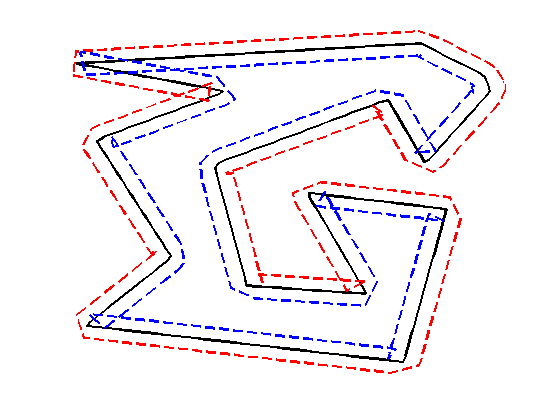## Evolution by Mean Curvature

A curve evolution is a series of curves $$s \mapsto \ga_s$$ indexed by an evolution parameter $$s \geq 0$$. The intial curve $$\ga_0$$ for $$s=0$$ is evolved, usually by minizing some energy $$E(\ga)$$ in a gradient descent $\frac{\partial \ga_s}{\partial s} = \nabla E(\ga_s).$

Note that the gradient of an energy is defined with respect to the curve-dependent inner product $\dotp{a}{b} = \int_0^1 \dotp{a(t)}{b(t)} \norm{\ga'(t)} d t.$ The set of curves can thus be thought as being a Riemannian surface.

The simplest evolution is the mean curvature evolution. It corresponds to minimization of the curve length $E(\ga) = \int_0^1 \norm{\ga'(t)} d t$

The gradient of the length is $\nabla E(\ga)(t) = -\kappa_\ga(t) n_\ga(t)$ where $$\kappa_\ga$$ is the curvature, defined as $\kappa_\ga(t) = \frac{1}{\norm{\ga'(t)}} \dotp{ t_\ga'(t) }{ n_\ga(t) } .$

Shortcut for normal times curvature $$\kappa_\ga(t) n_\ga(t)$$.

normalC = @(gamma)BwdDiff(tangent(gamma)) ./ abs( FwdDiff(gamma) );


Time step for the evolution. It should be very small because we use an explicit time stepping and the curve has strong curvature.

dt = 0.001 / 100;


Number of iterations.

Tmax = 3 / 100;
niter = round(Tmax/dt);


Initialize the curve for $$s=0$$.

gamma = gamma1;


Evolution of the curve.

gamma = gamma + dt * normalC(gamma);


To stabilize the evolution, it is important to re-sample the curve so that it is unit-speed parametrized. You do not need to do it every time step though (to speed up).

gamma = resample(gamma);


Exercice 1: (check the solution) Perform the curve evolution. You need to resample it a few times.

exo1;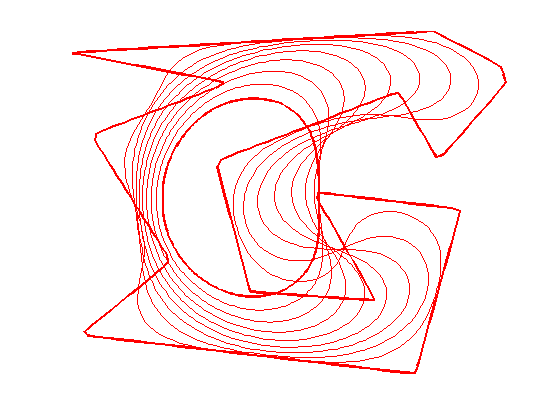## Geodesic Active Contours

Geodesic active contours minimize a weighted length $E(\ga) = \int_0^1 W(\ga(t)) \norm{\ga'(t)} d t,$ where $$W(x)>0$$ is the geodesic metric, that should be small in areas where the image should be segmented.

Create a synthetic weight $$W(x)$$.

n = 200;
nbumps = 40;
theta = rand(nbumps,1)*2*pi;
r = .6*n/2; a = [.62*n .6*n];
x = round( a(1) + r*cos(theta) );
y = round( a(2) + r*sin(theta) );
W = zeros(n); W( x + (y-1)*n ) = 1;
W = perform_blurring(W,10);
W = rescale( -min(W,.05), .3,1);


Display the metric.

clf;
imageplot(W);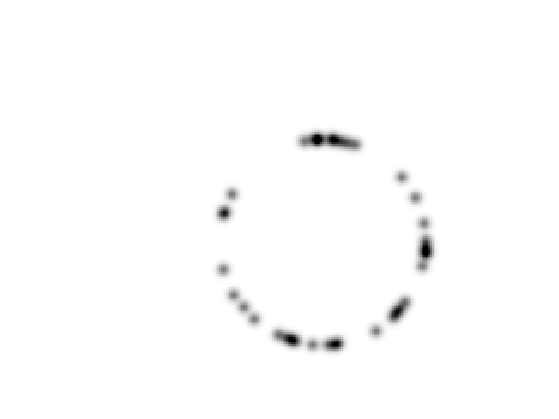Pre-compute the gradient $$\nabla W(x)$$ of the metric.

options.order = 2;
G = grad(W, options);
G = G(:,:,1) + 1i*G(:,:,2);


Shortcut to evaluate the gradient and the potential along a curve.

EvalG = @(gamma)interp2(1:n,1:n, G, imag(gamma), real(gamma));
EvalW = @(gamma)interp2(1:n,1:n, W, imag(gamma), real(gamma));


Create a circular curve $$\ga_0$$.

r = .98*n/2;
p = 128; % number of points on the curve
theta = linspace(0,2*pi,p+1)'; theta(end) = [];
gamma0 = n/2*(1+1i) +  r*(cos(theta) + 1i*sin(theta));


Initialize the curve at time $$t=0$$ with a circle.

gamma = gamma0;


For this experiment, the time step should be larger, because the curve is in $$[1,n] \times [1,n]$$.

dt = 1;


Number of iterations.

Tmax = 5000;
niter = round(Tmax/dt);


Display the curve on the back ground;

lw = 2;
clf; hold on;
imageplot(W);
h = plot(imag(gamma([1:end 1])),real(gamma([1:end 1])), 'r');
set(h, 'LineWidth', lw);
axis('ij');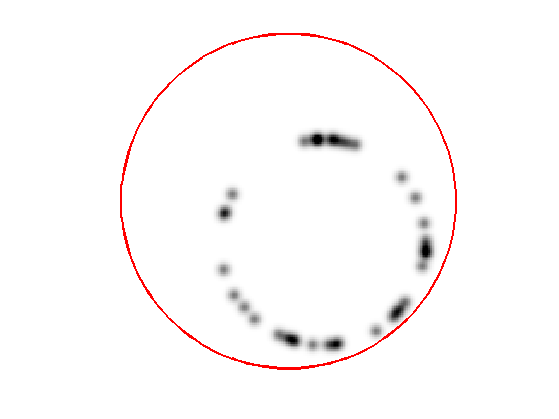The gradient of the energy is $\nabla E(\ga) = -W(\ga(t)) \kappa_\ga(t) n_\ga(t) + \dotp{\nabla W(\ga(t))}{ n_\ga(t) } n_\ga(t).$

Evolution of the curve according to this gradient.

N = normal(gamma);
g = - EvalW(gamma).*normalC(gamma) + dotp(EvalG(gamma), N) .* N;
gamma = gamma - dt*g;


To avoid the curve from being poorly sampled, it is important to re-sample it evenly.

gamma = resample( gamma );


Exercice 2: (check the solution) Perform the curve evolution.

exo2;## Medical Image Segmentation

One can use a gradient-based metric to perform edge detection in medical images.

Load an image $$f$$.

n = 256;
f = rescale( sum(load_image('cortex', n), 3 ) );


Display.

clf;
imageplot(f);An edge detector metric can be defined as a decreasing function of the gradient magnitude. $W(x) = \psi( d \star h_a(x) ) \qwhereq d(x) = \norm{\nabla f(x)}.$ where $$h_a$$ is a blurring kernel of width $$a>0$$.

Compute the magnitude of the gradient.

options.order = 2;
G = grad(f,options);
d = sqrt(sum(G.^2,3));


Blur it by $$h_a$$.

a = 3;
d = perform_blurring(d,a);


Compute a decreasing function of the gradient to define $$W$$.

d = min(d,.4);
W = rescale(-d,.8,1);


Display it.

clf;
imageplot(W);Number of points.

p = 128;


Exercice 3: (check the solution) Create an initial circle $$\gamma_0$$ of $$p$$ points.

exo3;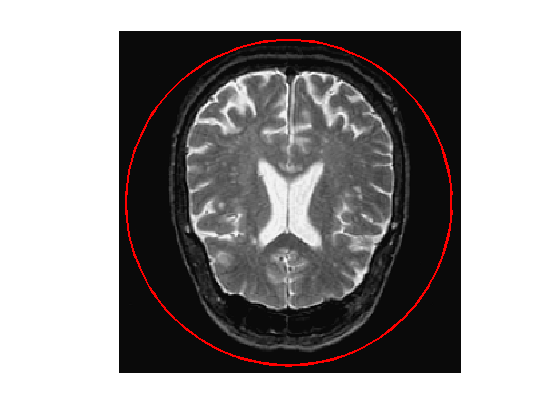Step size.

dt = 2;


Number of iterations.

Tmax = 9000;
niter = round(Tmax/dt);


Exercice 4: (check the solution) Perform the curve evolution.

exo4;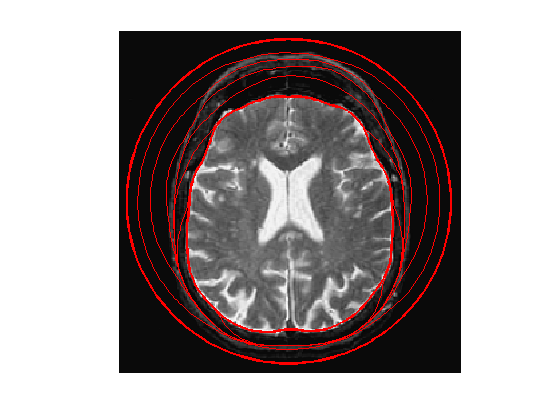## Evolution of a Non-closed Curve

It is possible to perform the evolution of a non-closed curve by adding boundary constraint $\ga(0)=x_0 \qandq \ga(1)=x_1.$

In this case, the algorithm find a local minimizer of the geodesic distance between the two points.

Note that a much more efficient way to solve this problem is to use the Fast Marching algorithm to find the global minimizer of the geodesic length.

Load an image $$f$$.

n = 256;
f = rescale( sum(load_image('cortex', n), 3 ) );
f = f(46:105,61:120);
n = size(f,1);


Display.

clf;
imageplot(f);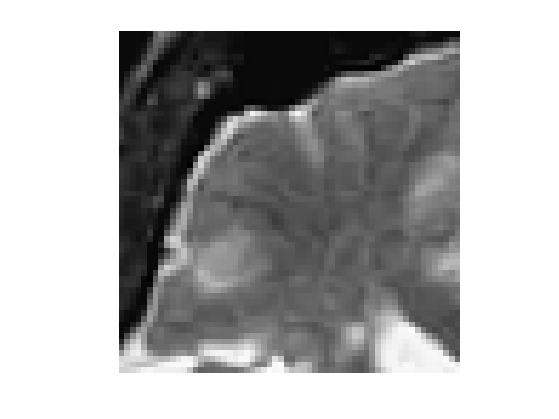Exercice 5: (check the solution) Compute an edge attracting criterion $$W(x)>0$$, that is small in area of strong gradient.

exo5;Start and end points $$x_0$$ and $$x_1$$.

x0 = 4 + 55i;
x1 = 53 + 4i;


Initial curve $$\ga_0$$.

p = 128;
t = linspace(0,1,p)';
gamma0 = t*x1 + (1-t)*x0;


Initialize the evolution.

gamma = gamma0;


Display.

clf; hold on;
imageplot(W);
h = plot(imag(gamma([1:end])),real(gamma([1:end])), 'r'); set(h, 'LineWidth', 2);
h = plot(imag(gamma([1 end])),real(gamma([1 end])), 'b.'); set(h, 'MarkerSize', 30);
axis('ij');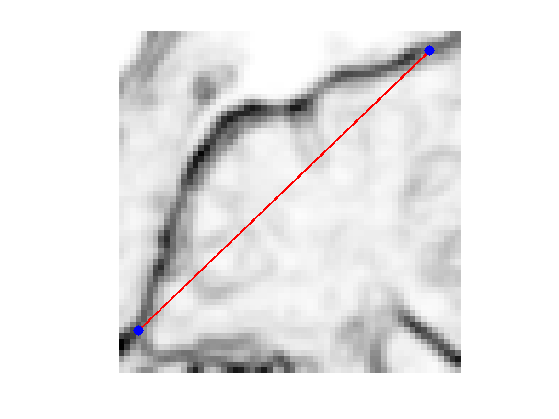Re-sampling for non-periodic curves.

curvabs = @(gamma)[0;cumsum( 1e-5 + abs(gamma(1:end-1)-gamma(2:end)) )];
resample1 = @(gamma,d)interp1(d/d(end),gamma,(0:p-1)'/(p-1), 'linear');
resample = @(gamma)resample1( gamma, curvabs(gamma) );


Time step.

dt = 1/10;


Number of iterations.

Tmax = 2000*4/7;
niter = round(Tmax/dt);


Exercice 6: (check the solution) Perform the curve evolution. Be careful to impose the boundary conditions at each step.

exo6;